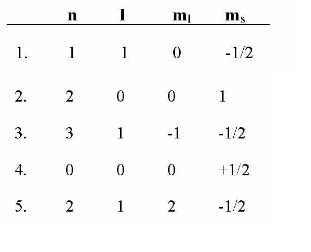# Problem: Which of the following is a possible set of quantum numbers?

###### FREE Expert Solution
92% (476 ratings)
###### FREE Expert Solution

We’re being asked to find which set of quantum numbers is possible

Recall that the quantum numbers that define an electron are:

• Principal Quantum Number (n): deals with the size and energy of the atomic orbital. The possible values for n are 1 to ∞.

• Angular Momentum Quantum Number (l): deals with the shape of the atomic orbital. The possible values for l are 0 to n – 1.

• Magnetic Quantum Number (ml): deals with the orientation of the atomic orbital in 3D space. The possible values for ml are the range of –l to +l.

• Spin Quantum Number (ms): deals with the spin of the electron. The possible values for ms are either –1/2 (spin down) or +1/2 (spin up).

92% (476 ratings)###### Problem Details

Which of the following is a possible set of quantum numbers?What scientific concept do you need to know in order to solve this problem?

Our tutors have indicated that to solve this problem you will need to apply the The Spin Quantum Number concept. You can view video lessons to learn The Spin Quantum Number. Or if you need more The Spin Quantum Number practice, you can also practice The Spin Quantum Number practice problems.

What is the difficulty of this problem?

Our tutors rated the difficulty ofWhich of the following is a possible set of quantum numbers?...as medium difficulty.

How long does this problem take to solve?

Our expert Chemistry tutor, Dasha took 2 minutes and 45 seconds to solve this problem. You can follow their steps in the video explanation above.

What professor is this problem relevant for?

Based on our data, we think this problem is relevant for Professor Covey's class at WEBER.